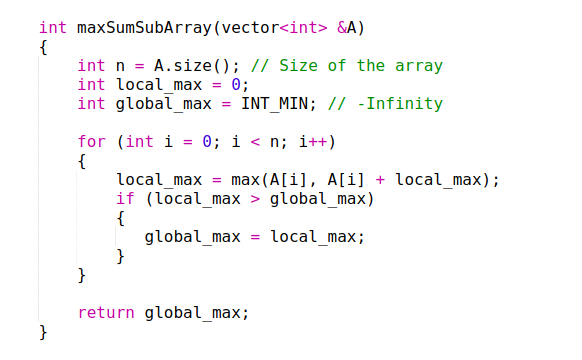If you’ve arrived here, it’s likely that you were looking for a solution to the “Maximum Subarray Problem” and came across Kadane’s Algorithm but couldn’t figure out how it worked. Or perhaps you’d had enough of utilising Kadane’s Algorithm as a “black box.” Or perhaps you wanted to learn more about dynamic programming. Or perhaps you simply want to learn about a new notion that will help you become a better programmer. You’ve arrived to the correct site for whatever reason.

To better grasp Kadane’s Algorithm, we’ll first go through a quick overview of Dynamic Programming. Then we’d look at the Maximum Subarray Problem, which is a well-known programming problem. We’d look at how a brute force technique can be used to tackle this problem, and then we’d try to refine our approach and come up with a better algorithm, dubbed Kadane’s Algorithm.

So, let’s get started.

## Dynamic Programming

Dynamic Programming is a method for solving a large problem by breaking it down into smaller subproblems, solving each of them once, and storing the results in a memory-based data structure (array, map, etc.). So, instead of recomputing the solution the next time the identical sub-problem arises, one simply looks up the already computed solution, saving computation time.

In this section, we would use the brute force approach discussed above again, but this time we would start backwards. How would that help? Let’s see.

We would start from the last element and calculate the sum of every possible subarray ending with element A[n-1], as shown in the figure below. Then, we would calculate the sum of every possible subarray ending with A[n-2], A[n-3] and so on up to A.

Backward Brute Force Approach: Iteration 0 (left) and Iteration 1 (right)

Now let’s focus on the subarrays ending with the element A (=-1) and A(=2) shown in the figure below.

From the figure above, we see that the local_maximum is equal to 3 which is the sum of the subarray [4, -1]. Now have a look at the subarrays ending with A. You’ll notice that these subarrays can be divided into two parts, the subarrays ending with A (highlighted with yellow) and the single element subarray A (in green).

Let’s say somehow I know the local_maximum. Then we see that to calculate the local_maximum, we don’t need to compute the sum of all subarrays ending with A since we already know the result from arrays ending with A. Note that if array [4, -1] had the maximum sum, then we only need to check the arrays highlighted with the red arrows to calculate local_maximum. And this leads us to the principle on which Kadane’s Algorithm works.

local_maximum at index i is the maximum of A[i] and the sum of A[i] and local_maximum at index i-1.

This way, at every index i, the problem boils down to finding the maximum of just two numbers, A[i] and (A[i] + local_maximum[i-1]). Thus the maximum subarray problem can be solved by solving these sub-problems of finding local_maximums recursively. Also, note that local_maximum would be Aitself.

Using the above method, we need to iterate through the array just once, which is a lot better than our previous brute force approach. Or to be more precise, the time complexity of Kadane’s Algorithm is O(n).

Finally, let’s see how this all would work in code.

## Code Walkthrough

Below is a very much self-explanatory implementation (in C++) of a function which takes an array as an argument and returns the sum of the maximum subarray.Note that instead of using an array to store local_maximums, we are simply storing the latest local_maximum in an int type variable ‘local_max’ because that’s what we need to calculate next local_maximum. Also, as we are using a variable ‘global_max’ to keep track of the maximum value of local_maximum, which in the end comes out to be the required output.

## Conclusion

This algorithm can be viewed as a simple example of dynamic programming because of the way it uses optimal substructures (the maximum subarray ending at each position is calculated in a simple way from a related but smaller and overlapping subproblem: the maximum subarray ending at the previous position). With a runtime of O(n), Kadane’s approach can discover the largest sum of a contiguous subarray in an array (n).